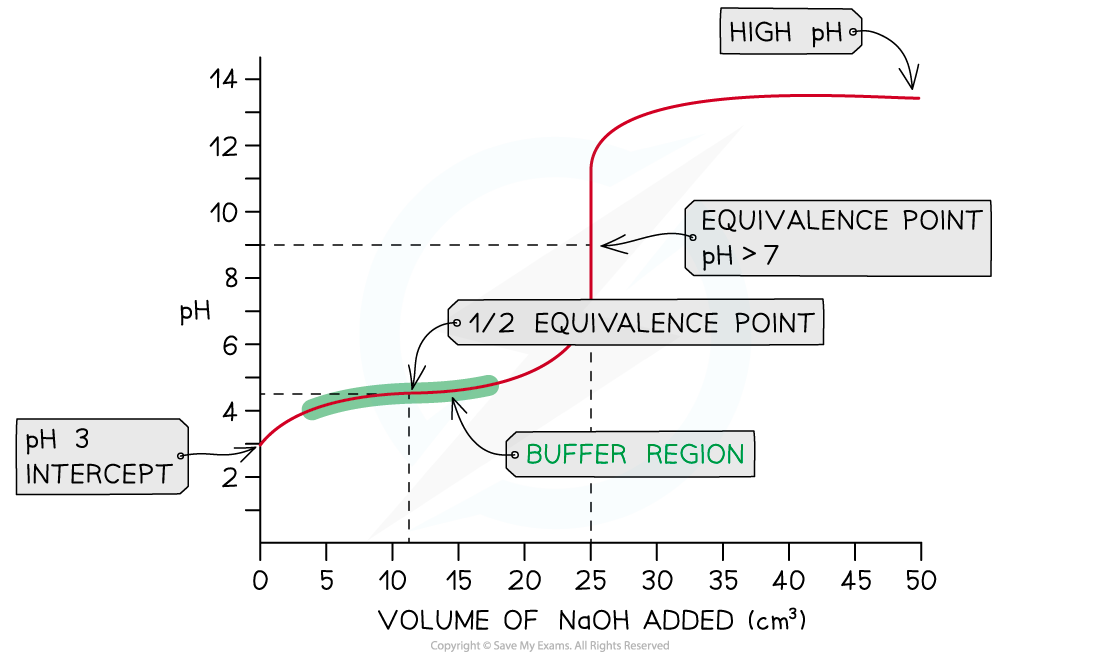# Edexcel A Level Chemistry:复习笔记5.2.8 Weak Acid - Strong Base Titration

### Weak Acid - Strong Base Titration

• In this example, strong sodium hydroxide, NaOH (aq), is being added to weak ethanoic acid, CH3COOH (aq)

NaOH (aq) + CH3COOH (aq) → CH3COONa (aq) + H2O (l)

• The pH on the intercept on the y axis starts at roughly 3 due to the relative strength of the ethanoic acid
• The initial rise in pH is steep as the neutralisation of the weak acid by the strong base is rapid
• Ethanoate ions (conjugate base to ethanoic acid) are formed which then creates a buffer
• A buffer consists of a weak acid and its conjugate base or a weak base and its conjugate acid

• At this point, the buffer formed will resist changes in pH so the pH rises gradually as shown in the buffer region
• The half equivalence point is the stage of the titration at which exactly half the amount of weak acid has been neutralised
• [CH3COOH (aq)] = [CH3COO- (aq)]
• At this point, it is important to note that the pKa of the acid is equal to the pH
• pKa = pH at half equivalence
• The equivalence point in a weak acid - strong base titration is above 7Weak acid - strong base pH curve